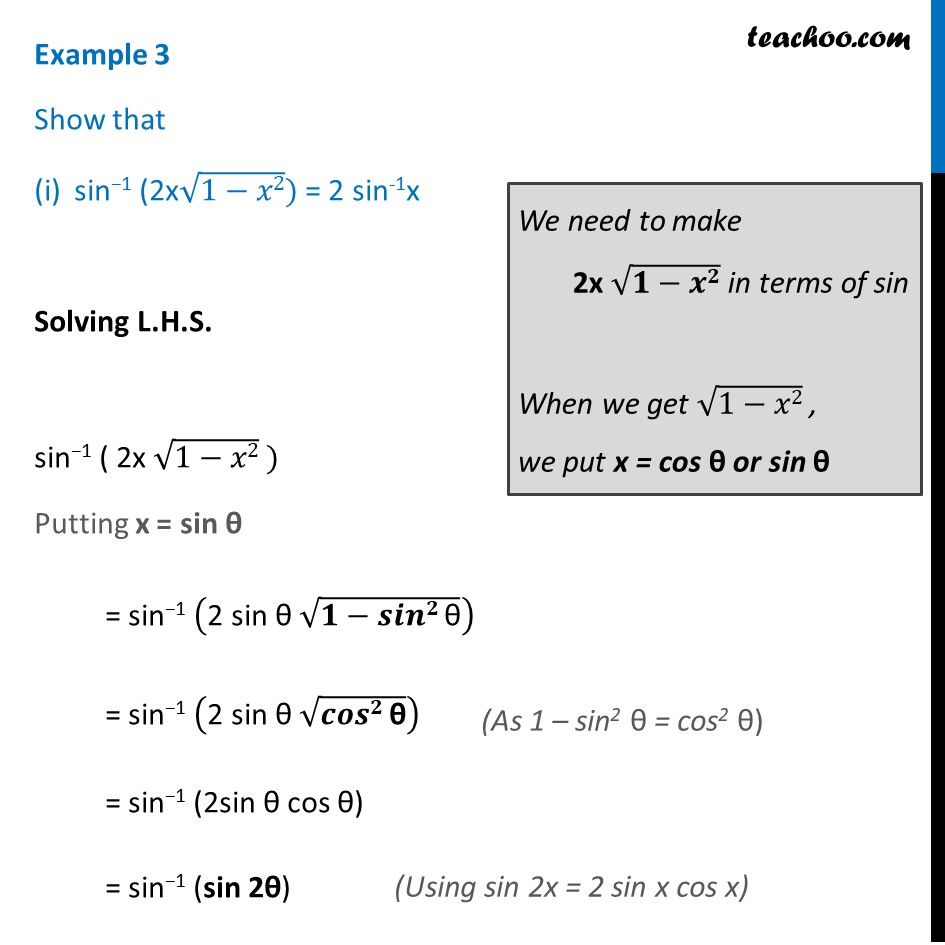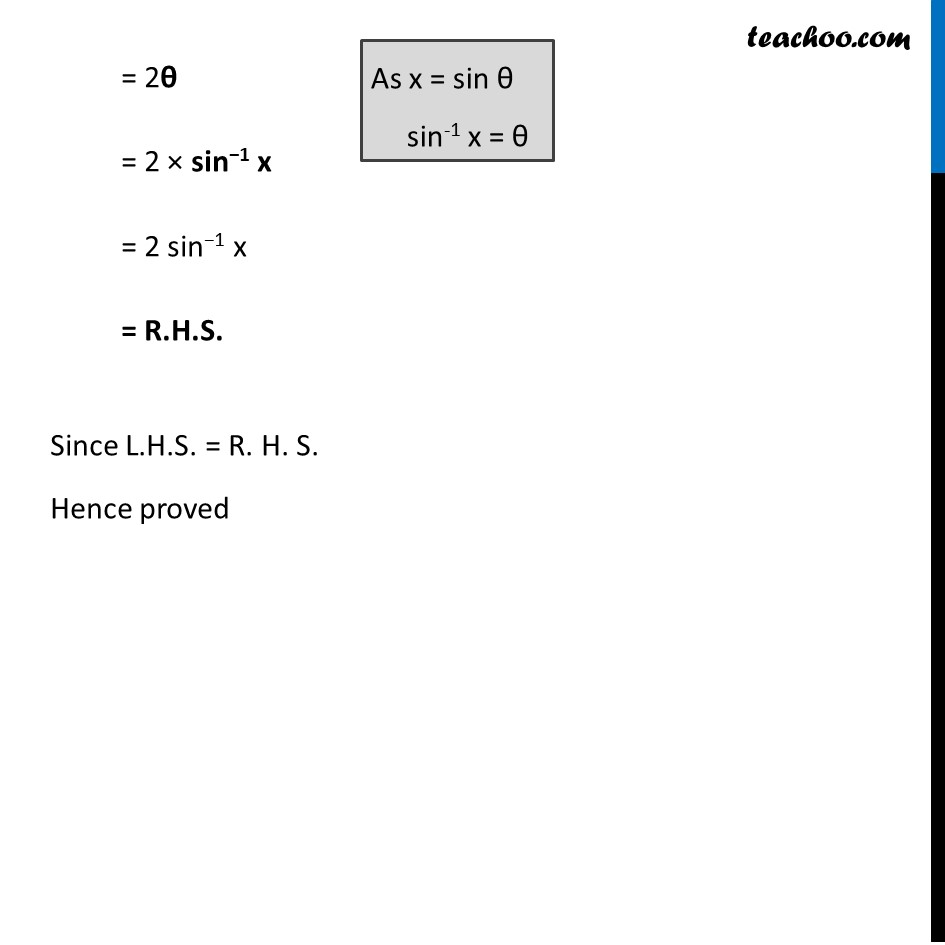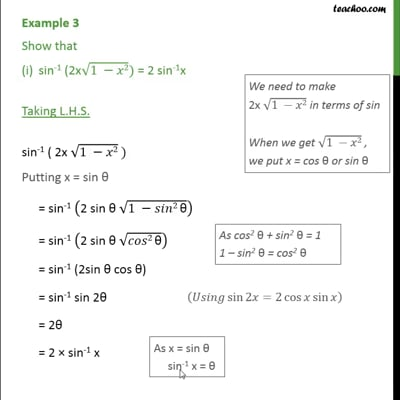Formulae based

Chapter 2 Class 12 Inverse Trigonometric Functions
Concept wiseThis video is only available for Teachoo black users

Introducing your new favourite teacher - Teachoo Black, at only ₹83 per month

### Transcript

Example 3 Show that sin−1 (2x√(1−𝑥2)) = 2 sin-1x Solving L.H.S. sin−1 ( 2x √(1−𝑥2) ) Putting x = sin θ = sin−1 ("2 sin θ " √(𝟏−𝒔𝒊𝒏𝟐" θ" )) = sin−1 ("2 sin θ " √(𝒄𝒐𝒔𝟐" θ" )) = sin−1 (2sin θ cos θ) = sin−1 (sin 2θ) (As 1 – sin2 θ = cos2 θ) (Using sin 2x = 2 sin x cos x) (Using sin 2x = 2 sin x cos x) = 2θ = 2 × sin−1 x = 2 sin−1 x = R.H.S. Since L.H.S. = R. H. S. Hence proved As x = sin θ sin-1 x = θ